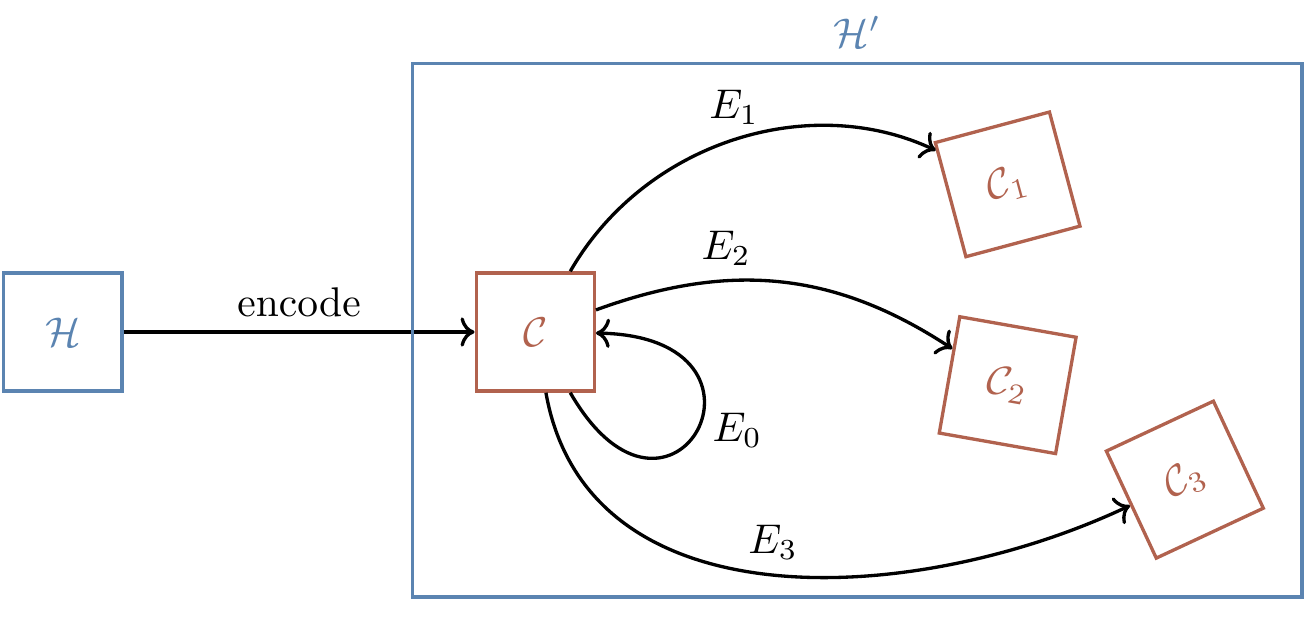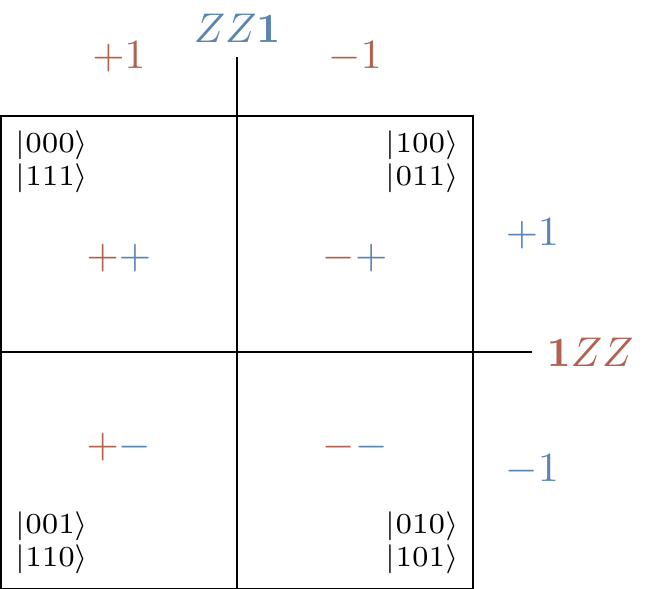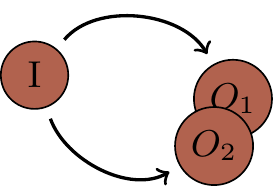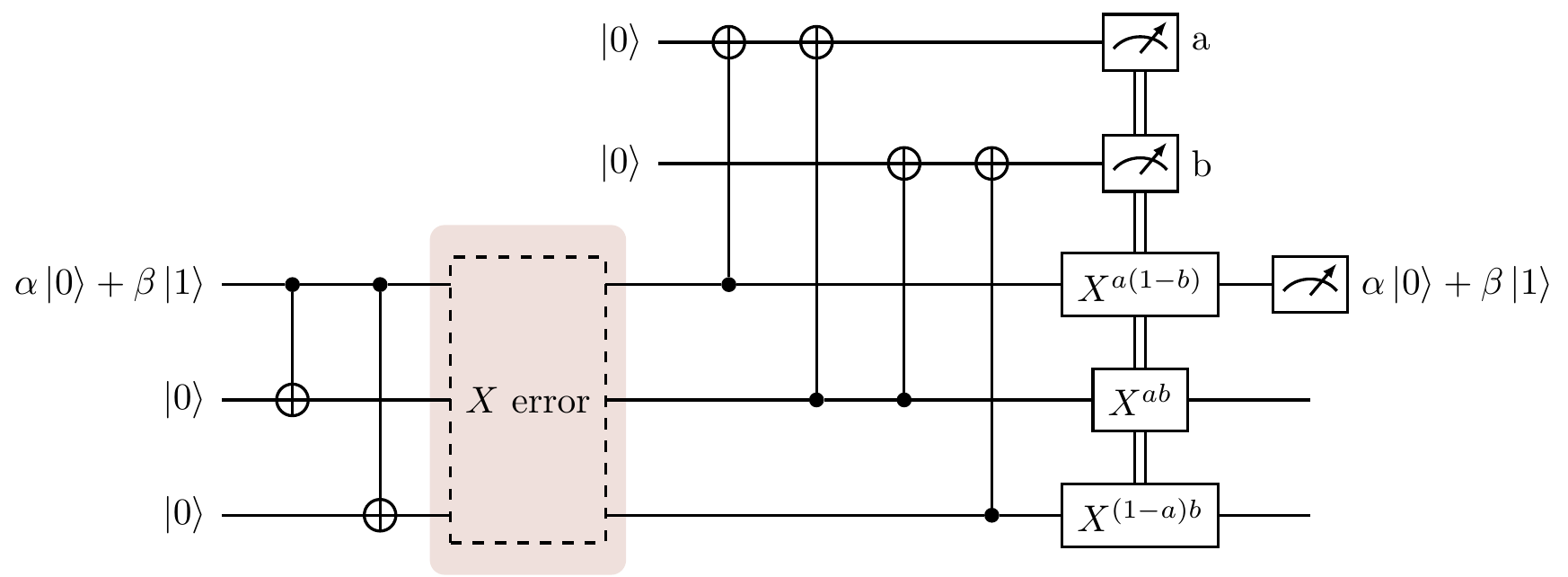## 13.7 Correcting bit-flips

In order to protect a qubit against bit-flips (thought of as incoherent X rotations), we rely on the same classical repetition code as in Section 13.6, but both encoding and error correction are now implemented by quantum operations.268 Let’s return to the example we introduced in Section 13.1. We take a qubit in some unknown pure state \alpha|0\rangle+\beta|1\rangle and encode it into three qubits, introducing two auxiliary qubits:Mathematically, this is an isometric269 embedding of a two-dimensional space into an eight-dimensional one.

Now suppose that one qubit is flipped, say, the second one. The encoded state then becomes \alpha|010\rangle+\beta|101\rangle. Decoding requires some care: measuring the three qubits directly would destroy the superposition that we are working so hard to protect. So instead we introduce two ancilla qubits, both in state |0\rangle, and apply the following circuit:This decoding circuit is exactly the same as the ones for measuring the Pauli stabilisers ZZ\mathbf{1} and \mathbf{1}ZZ (as described in Section 7.4).

Measuring the two ancilla qubits gives us what is known as the error syndrome, which tells us how to correct the three qubits (known as the data qubits) of the code. The theory behind this works as follows:

• if the first and second (counting from the top) data qubits are in the same state then the first ancilla will be in the |0\rangle state; otherwise the first ancilla will be in the |1\rangle state
• if the second and third data qubits are in the same state then the second ancilla will be in the |0\rangle state; otherwise the second ancilla will be in the |1\rangle state.

So the four possible error syndromes each indicate a different scenario:270

• |00\rangle: no error
• |01\rangle: bit-flip in the first data qubit
• |10\rangle: bit-flip in the second data qubit
• |11\rangle: bit-flip in the third data qubit.

In our example, the error syndrome is |11\rangle, and so we know that the first and second qubits differ, as do the second and third. This means that the first and third must be the same, and the second suffered the bit-flip error. Knowing the error, we can now fix it by applying an X gate to the second qubit. The final result is the state \alpha|000\rangle+\beta|111\rangle, which is then turned into (\alpha|0\rangle+\beta|1\rangle)|00\rangle by running the mirror image of the encoding circuit:It is also important to note that the actual error correction can be implemented by a single unitary operation U_c on the five total qubits, with \begin{aligned} U_c &= \big(|0\rangle\langle 0|\otimes|0\rangle\langle 0|\big)\otimes(\mathbf{1}\mathbf{1}\mathbf{1}) \\&+ \big(|0\rangle\langle 0|\otimes|1\rangle\langle 1|\big)\otimes(\mathbf{1}\mathbf{1}X) \\&+ \big(|1\rangle\langle 1|\otimes|0\rangle\langle 0|\big)\otimes(X\mathbf{1}\mathbf{1}) \\&+ \big(|1\rangle\langle 1|\otimes|1\rangle\langle 1|\big)\otimes(\mathbf{1}X\mathbf{1}). \end{aligned}Figure 13.8: The quantised version of the classical [3,1,3]-code. If at most one bit-flip error occurs in the shaded region (which denotes the part where we transmit over a noisy channel), then this circuit perfectly corrects it, resulting in the successful transmission of the state \alpha|0\rangle+\beta|1\rangle.

1. All the codes we will study have encoding circuits that can be constructed out of controlled-\texttt{NOT} and Hadamard gates: we are dealing with Clifford circuits (recall Section 7.7).↩︎

2. Recall that an isometry is the generalisation of a unitary but where we are also allowed to bring in additional qubits.↩︎

3. Again, for now we are assuming that at most one bit-flip error occurs.↩︎